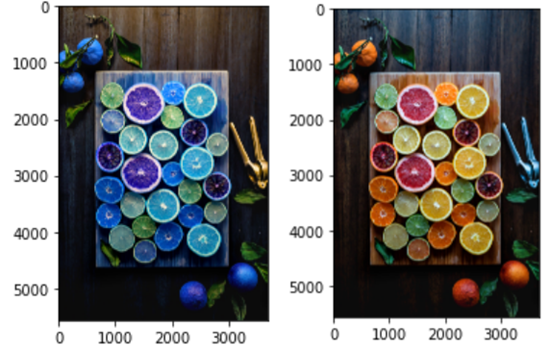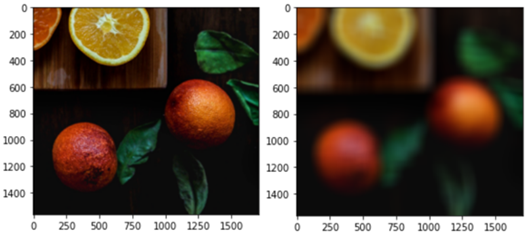# Python OpenCV Image Processing – Resize, Blend, Blur, Threshold, Convert

Rate this post

This tutorial is an introduction to the OpenCV library. Learn how to convert color channels, resize, blend, blur, and threshold images in Python.

The OpenCV  library contains most of the functions we need for working with images. Handling images in programming requires a different intuition than handling text data. An image is made up of pixels. It looks like a spreadsheet full of cells with numerical values when zoomed in. Each pixel usually contains a value ranging between 0 to 255. The value indicates the degree of brightness for the color it is assigned to. So, how do we work with images in Python? We first need to load them as NumPy arrays, converting all image pixels into numerical values. Only then we can use different computer vision techniques to manipulate them.

In this article, we are going to get our hands dirty experimenting with images using OpenCV. We will look at techniques like color conversion, resizing, blending, blurring, and thresholding. Getting your image data right is a half-way success for a useful machine learning model. Intrigued? Let’s get started.

## Install and Import Required Modules

For this tutorial, we need to install the OpenCV, NumPy, and Matplotlib modules. NumPy is used to manipulate image arrays. Matplotlib is used to display images for comparing the “before and after”. Feel free to clone the GitHub repo of this tutorial.

First, create a virtual environment for this project. Then, install the mentioned modules in a Jupyter notebook:

```!pip install opencv-python
!pip install numpy
!pip install matplotlib```

No surprise here — the installation should be straightforward and fast. Now execute the following lines of code in your notebook:

```import cv2
import numpy as np
import matplotlib.pyplot as plt
%matplotlib inline
```

Note that the %matplotlib inline magic command is exclusive for Jupyter notebooks. It is not required in a Python script. It sets the backend of the Matplotlib module to display figures inline and not on a separate window.

## Load Image and Convert Color Channels

To load an image in the notebook, we use the imread method of the OpenCV module. By default, the method loads an image in color. To load a greyscale image, we need to supply a second parameter of “0’” to the method:

```img_greyscale = cv2.imread('./photo.jpg', 0)
img_greyscale.shape
img.shape
```

Note that the images are loaded as NumPy arrays – one greyscale and another one in color. The shape method returns (5563, 3709) for the variable img_greyscale and (5563, 3709, 3) for img. The method returns information in the form of (height, width, channel). Both the variables have the same height and width values. But img_greyscale consists of only one channel (one color) while img has three.

By default, the imread method loads an image with a color order of blue, green, red. It is not the usual red, green, blue. In case you ever wonder why your images look weird in OpenCV, it is that. To display an image, use the imshow method of the Matplotlib module as follows:

`plt.imshow(img)`Figure 1: (Left) An image with (Blue, Green, Red) color channels. (Right) The same image with (Red, Green, Blue) color channels.

Figure 1 shows how different an image can look when its color channels are mixed up. Matplotlib displays the red channel as blue for the image on the left. To fix this, we can use the OpenCV cvtColor method to convert the color channels from (B, G, R) to (R, G, B), as follows:

`img_RGB = cv2.cvtColor(img, cv2.COLOR_BGR2RGB)`

The color-corrected image is shown on the right side of Figure 1. We will use the RGB image as an example in the latter sections. But using RGB images is not a requisite — feel free to use the BGR image if you prefer. Make sure to pick the correct channels in operation.

## Resize Image

Quiz time: which OpenCV method should you use to resize an image? You guessed it — the resize method. It takes an image and an image dimension as parameters. The following code resizes the image to be half its original size:

```width = int(img_RGB.shape / 2)
height = int(img_RGB.shape / 2)

img_RGB_smaller = cv2.resize(src=img_RGB, dsize=(width, height))
img_RGB_smaller.shape
```

Note that you can supply any positive integer values to the dsize parameter of the resize method. Yet, it is a good practice to use a scale factor to keep the original aspect ratio of the image. The code shown takes the width and height values of the original image and divides them by two. The output of the img_RGB_smaller.shape is (2781, 1854, 3), which is 50% smaller than its original size, (5563, 3709, 3). You can also make the image larger by multiplying its width and height by two, as follows:

```width = int(img_RGB.shape * 2)
height = int(img_RGB.shape * 2)

img_RGB_bigger = cv2.resize(src=img_RGB, dsize=(width, height))
img_RGB_bigger.shape
```

That creates an image of size (11126, 7418, 3). Feel free to be creative with the image dimension definitions. Figure 2 shows the resized images. Both look the same because their aspect ratios are retained. Note the differences in their width and height axes instead.Figure 2: (Left) An image resized to half its original size. (Right) The same image resized to double its original size.

## Blend Images

Image blending means combining two images with shared transparency. We want two images to “blend into” each other as one image. For this, we need to load another image to our notebook:

```img_overlay = cv2.imread('./photo-overlay.jpg')
img_overlay.shape
```

All images used in this code project can be found at Unsplash.com. The second image is loaded as variable img_overlay with dimensions (2000, 1800, 3). Images must have the same size for image blending. As img_overlay is of a different size than the first image, we need to resize it to match the size of the first image:

```img_overlay = cv2.resize(img_overlay, (img_RGB.shape, img_RGB.shape))
img_overlay.shape
```

Note that the dsize parameter takes a value in the form of (width, height), not (height, width). Thus, we enter (img_RGB.shape, img_RGB.shape) as the parameter instead of the other way round. Now, the output of img_overlay.shape should show the same size as img_RGB, which is (5563, 3709, 3). Enter the following code to blend both the images together:

`blended = cv2.addWeighted(src1=img_RGB, alpha=0.3, src2=img_overlay, beta=0.7, gamma=0)`

The addWeighted method of OpenCV combines the images with a “transparency weightage”. The src1 parameter takes the background image and the src2 the foreground image. The alpha parameter sets the transparency of src1 and the beta of src2. Both alpha and beta can take values ranging from 0 to 1 and should both add up to 1. A value closer to 0 indicates more transparency. A value closer to 1 indicates more opaqueness. The gamma parameter sets the brightness of the output image. Figure 3 shows the before and after of the image blending operation.Figure 3: (Top left) The background image. (Top right) The foreground image. (Bottom) The blended image.

## Blur Image

Here, we crop out a smaller section of an image to better notice the image blurring operation. Taking img_RGB, we copy its bottom right part as img_small using NumPy array slicing:

`img_small = img_RGB[4000:, 2000:]`

That will create a smaller image of size (1563, 1709, 3). There are various image blurring functions in the OpenCV module. For example, average blurring, median blurring, and Gaussian blurring. They differ in their mathematical operations and outcomes. For the sake of simplicity, we use the basic average blurring function in this tutorial. Enter the following line of code in your notebook:

`blurred = cv2.blur(src=img_small, ksize=(100, 100))`

You must have familiarized yourselves with the OpenCV parameters by now. Otherwise, press the SHIFT + TAB buttons to view any function description. The ksize parameter of the blur method defines the dimensions of the filter kernel. A kernel is like a paintbrush or sponge that you use to “smudge” the original image and make it blurry. The ksize parameter is the width and height of the sponge that you want to use – in this case, 100 x 100. Figure 4 shows the cropped image with its blurred after effect.Figure 4: (Left) A cropped image. (Right) The same image blurred with a kernel sized (100, 100).

## Threshold Image

Image thresholding turns a greyscale image into either black or white pixels. You might be asking: what’s the need for blurring and thresholding images? The answer is: so that computational models can perceive image data better. Take edge detection as an example: we want to blur or smooth object edges so that there will be less noise. And we want to threshold images so object boundaries can be defined better.

For thresholding, we use img_greyscale instead of the coloured image. Enter the following one-liner in your notebook:

`ret, thresh1 = cv2.threshold(src=img_greyscale, thresh=127, maxval=255, type=cv2.THRESH_BINARY)`

The threshold method takes a greyscale image as its src parameter. The thresh parameter is the cutting-point for the black/white pixel decision. Any pixel value lower than the thresh value will be assigned 0. Any pixel value above the thresh value will be assigned 1. That creates the black-or-white contrast. As the image has its values ranged from 0 to 255, we assign the maxval (largest value) parameter as 255. The type parameter defines the kind of threshold we want. THRESH_BINARY converts all shades of grey in the image into either black or white. Figure 5 shows a greyscale image with its outcome after the thresholding operation.